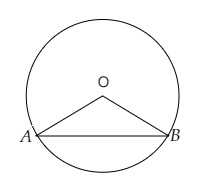# In a circle of radius $6 \mathrm{~cm}$, a chord of length $10 \mathrm{~cm}$ makes an angle of $110^{\circ}$ at the centre of the circle. Find the length of the arc $A B$.

Given:

Radius of the circle $r=6 \mathrm{~cm}$.

Length of the arc $l=10 \mathrm{~cm}$.

Angle subtended at the centre $=110^{\circ}$.

To do:

We have to find the length of the arc $A B$.

Solution:Let $OA$ and $OB$ are the radii of the circle and $AB$ the chord.

We know that,

Length of an arc subtending an angle $\theta$ at the centre is $2 \pi r (\frac{\theta}{360^{\circ}})$.

Therefore,

Length of the arc $=2 \pi r \times \frac{\theta}{360^{\circ}}$

$=2 \times 3.14 \times 6 \times \frac{110^{\circ}}{360^{\circ}} \mathrm{cm}$

$=12 \times 3.14 \times \frac{11}{36} \mathrm{cm}$

$=\frac{34.54}{3} \mathrm{cm}$

$=11.51 \mathrm{~cm}$

The length of the arc is $11.51\ cm$.

Updated on: 10-Oct-2022

38 Views